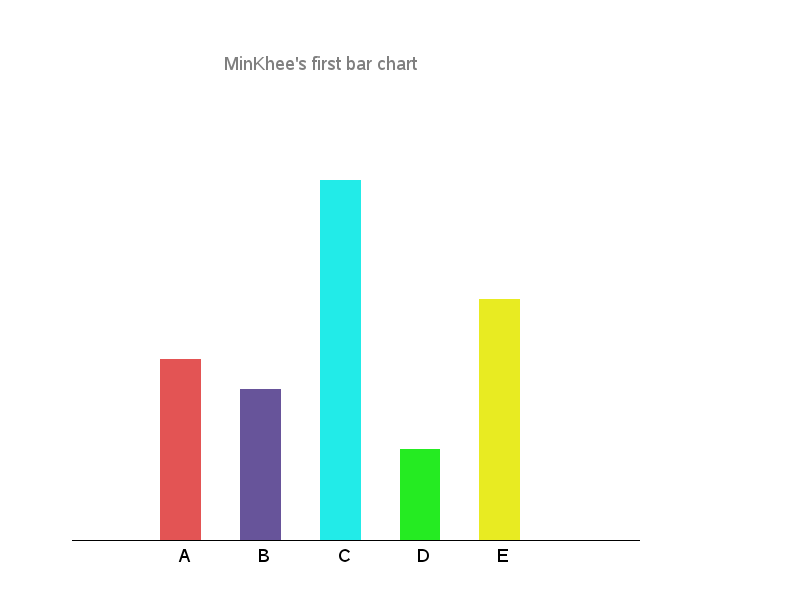## Draw bar chart by using sas ganno

Hi! I want to draw a bar chart using fully customized annotate, using axis order 2, only the data area. The chart must drawn without using proc gchart.

Here is the data:

A 70  cxe35454

B 60  cx67549A

C 130 cx22ebe8

D 40  cx25eb22

E 90  cxe8eb22

And here is the results that I want:

data yoyo;

length function color \$8 text \$30;

retain xsys ysys '2' hsys '3' ;

function='move';x=20;y=10;

output;

function='bar';x=25;y=40;color='CXE35454';style='solid';line=0;

output;

function='move';x=30;y=10;

output;

function='bar';x=35;y=35;color='CX67549A';

output;

function='move';x=40;y=10;

output;

function='bar';x=45;y=70;color='CX22EBE8';

output;

function='move';x=50;y=10;

output;

function='bar';x=55;y=25;color='CX25EB22';

output;

function='move';x=60;y=10;

output;

function='bar';x=65;y=50;color='CXE8EB22';

output;

function='label';x=23;y=8;text='A';position='5';color='black';size=3;style='calibri';

output;

function='label';x=33;y=8;text='B';

output;

function='label';x=43;y=8;text='C';

output;

function='label';x=53;y=8;text='D';

output;

function='label';x=63;y=8;text='E';

output;

function='label';x=40;y=90;text="MinKhee's first bar chart";position='5';color='CX808080';style='calibri';size=3;

output;

function='move';x=9;y=9.8;

output;

function='draw';x=80;y=9.8;color=black;size=0.1;line=0;

output;

run;

title j=center

font= 'calibri' color=CX808080 height=45pt "MinKhee's first bar chart ";

proc ganno annotate=yoyo;

run;

quit;

Hope you all understand what I meant3 REPLIES 3

## Re: Draw bar chart by using sas ganno

Sorry, no its not clear.  I do not understand why you are restricted to using one procedure, use the right tool for the job.  From my side I would recommend that you look to using sgplot or graph template language.  These are the newest technology for creating graphical outputs and are more powerful and easy to use than previous installments.  If you need help on them Sanjay has an excellent blog showing examples:

http://blogs.sas.com/content/graphicallyspeaking/

## Re: Draw bar chart by using sas ganno

I think you are looking for the annotate macros:

%annomac; /* before your data set to make the macros available*/

and use %bar to draw the bars and %label to create the labels but your X Y coordinates need to be in the data or you need to provide the logic to assign them. Maybe additional variable retained and incremented by you for x.

Note that the annotate macros may update internal variables named XLAST, YLAST, XLSTT, YLSTT that help keep track of the current drawing position.

## Re: Draw bar chart by using sas ganno

To use xsys & ysys = '2', I think you need to have an actual chart, so that you have an axis for your coordinates to be inside.

In your case, I'd just use xsys & ysys = '3', and use Proc Gslide (I always use that for a 'blank' page, instead of Proc Ganno - just my personal preference).

When I change to the following ...

retain xsys '3' ysys '3' hsys '3' ;

proc gslide anno=yoyo;
run;

I get your custom-drawn bar chart as follows:Discussion stats
• 3 replies
• 957 views
• 0 likes
• 4 in conversation